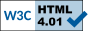## PCPs and Limits of approximation algorithms (two module course) - Winter/Summer Semester (2014-15)

Instructor:

#### Problem Sets

1. Module - I
1. Problem Set 1 [pdf]
2. Module - II
1. Problem Set 1 [pdf]

### Module I: PCP constructions (10 Feb - 20 Mar)

Time: Thurs 14:30-17:00
Location: D-405

#### Lectures

1. Feb 10: What is a proof?
Administrivia, What is a proof? Godel, Turing to today. Proof Systems, local vs. global, Interactive proof systems, MIP, PCP.
Ref: [ Har2 (Lecture 4), O'D ]

2. Feb 13: The PCP Theorem
The PCP Theorem and its strengthenings, Sliding Scale Conjecture
Ref: [ Har2 (Lecture 4)]

3. Feb 17: Linearity Testing
Blum-Luby-Rubinfeld's homomorphism testing, Coppersmith's example and the BLR analysis, Linearity testing over GF(2) using Fourier Analysis.
Ref: [ Har2 (Lecture 5 and 6)]

4. Feb 20: Exponential sized PCPs via linearity testing - I
Coding theory - primer, Walsh-Hadamard code (local testability, local decodability), NP \subseteq PCP[poly, O(1)].

5. Feb 23: Exponential sized PCPs via linearity testing - II
NP \subseteq PCP[poly, O(1)], PCP of proximity, Reed Muller codes.

6. Feb 28: Low-degree testing - I
Raz-Safra Plane-point test, properties of planes' oracle.

7. Feb 28: Low-degree testing - II
Raz-Safra Plane-point test and its analysis.

8. Mar 6: PCPs based on low-degree testing
PCPs for zero-on-subcube using low degree testing, NP \subseteq PCP[O(log n), polylog n].

9. Mar 10: Proof Composition
PCPs of proximity, robust PCPs, Proof composition, proof of the PCP Theorem.

10. Mar 15: Parallel Repetition - I

11. Mar 17: Parallel Repetition - II

12. Mar 15: Parallel Repetition - III

### Module II: Hardness of approximation (14 May - 25 Jun)

Time: Tue-Thurs 14:00-15:30
Location: A-212

#### Lectures

1. May 14: Approximation algorithms and introduction to inapproximability
Administrivia, Approximation of NP-complete combinatorial optimization problems; Approximability of Vertex Cover, TSP; Introduction to inapproximability, and the PCP Theorem.
Ref: [ Har2 (Lecture 1 and 3)]

2. May 19: Inapproximability of clique
The PCP Theorem - two views (inapproximability and proof checking); Inapproximability of clique
Ref: [ Har2 (Lecture 4)]

3. May 21: Inapproximability of MAX-3LIN2 - I
Gap preserving reductions; MAX-3LIN2 vs. MAX-3SAT; Introduction to Fourier Analysis;
Ref: [ Har2 (Lecture 6)]

4. May 25: Linearity testing
Linearity testing and Fourier based analysis of linearity testing
Ref: [ Har2 (Lecture 6)]

5. May 28: Label cover and long code
Label cover, parallel repetition, long code, long code test.
Ref: [ Har2 (Lecture 5 and 11)]

6. Jun 2: Inapproximability of MAX-3LIN2 - III
Complete proof of hardness of MAX-3LIN2; Introduction to gadget reuctions.
Ref: [ Har2 (Lecture 11)]

7. Jun 4: Optimal Gadget Reductions
Ref: [ Sud (Lecture 22)]

8. Jun 9: Inapproximability of Set-Cover - I
Set Cover, Greedy algorithm, (m,l)-set systems, Lund-Yannakakis reduction
Ref: [ GO (Lecture 14) ]

Jun 11: No class (due to Madhu Sudan's talk on PCPs)

9. Jun 16: Inapproximability of Set-Cover - II
(m,l)-set systems, Lund-Yannakakis reduction, Feige's improvement
Ref: [ GO (Lecture 14), Sud (Lecture 24), Fei ]

10. Jun 18: MAXCUT and its approximability
MAXCUT, SDP relaxation, Goeman-Williamson rounding algorithm; Introduction to Unique Games.
Ref: [ Har2 (Lecture 3 and 12)]

#### Tentative list of topics (for future lectures)

• Unique games and hardness of MAXCUT
• UG hardness of vertex cover
• Integrality ratio
• ....

#### Requirements (for each module)

Students taking the course for credit will be expected to:

• Attend lectures
• Do problem sets (2 problems sets)

#### References

1. [Fei] Uriel Feige. A Threshold of ln n for Approximating Set Cover. J. ACM, 45(4): 634-652, 1998.
(Preliminary version in 28th STOC, 1996). doi:10.1145/285055.285059.
2. [GO] A course on PCPs (offered by Venkatesan Guruswami and Ryan O'Donnell) at the University of Washington, Seattle:
CSE 533: The PCP Theorem and Hardness of Approximation (Autumn 2005).
3. [Har1] A course on PCPs (offered by Prahladh Harsha) at the University of Chicago:
CMSC 39600: PCPs, codes and inapproximability (Autumn 2007).
4. [Har2] A course on PCPs (offered by Prahladh Harsha) at TIFR and IMSc:
Limits of approximation algorithsm: PCPs and Unique Games.
5. [O'D] Ryan O'Donnell, A History of the PCP Theorem.
6. [Sud] A course on Approximability of Optimization Problems (offered by Madhu Sudan) at MIT:
6.893: Approximability of Optimization Problems (Fall 1999).

This page has been accessed at leasttimes since February 20, 2015.

 Prahladh Harsha